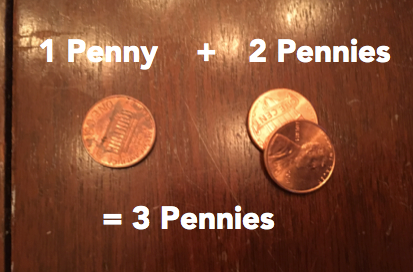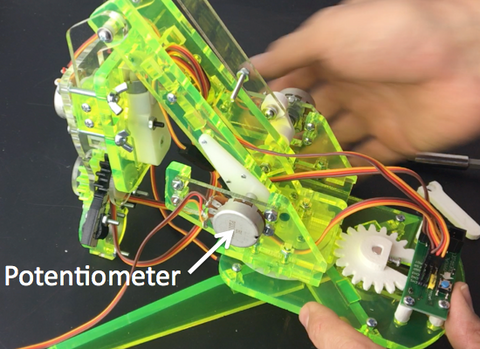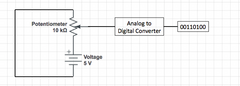# What do Analog and Digital Mean Anyway?

We all talk about "digital" technology, but do you really know what it means? Most people would say that "digital" is synonymous with "electronic" or "computerized", but in fact there is such a thing as an analog computer and a digital mechanical device, and it's not always clear which one is better.

Ok, then what does "analog" actually mean?

According to Dictionary.com Analog, when used as an adjective means:

1. of or relating to a mechanism that represents data by measurement of a continuous physical variable, as voltage or pressure.

We can demonstrate analog arithmetic using measuring cups. Imagine how we could add 1 + 2 using water. First, fill one measuring cup with 1 cup of water. Next fill a second measuring cup with 2 cups of water. Dump them both into a third cup, and presto! You've just added 1 + 2 to make 3!There's even an advantage to doing math this way. Analog has a capability that digital mechanisms don't. An analog measurement can be infinitesimally small. If you were able to look closely enough you'd be able to tell the difference between 1 cup, 1.000001 cups, or even 1.00000000...001 cups all the way out to infinity zeros. Digital measurements are always limited to the defined size of a "bit", which we'll get to in a moment.

Unfortunately, Analog's biggest advantage is also it's biggest flaw. Did you really add 1 cup plus 2 cups just now? Nope. You added something close, but not quite exactly what you were after. Perhaps 1.0001 cups, or .999 cups. Because analog measurements can be infinitesimally small they are also NEVER perfectly accurate.

Ok, then what's Digital?

One of many definitions of digital on Dictionary.com is:

involving or using numerical digits expressed in a scale of notation, usually in the binary system, to represent discretely all variables occurring in a problem.

This means that the accuracy of your digital measurement is limited to the number of discrete "bits" that you have. Let's try it. Imagine you want to add 1 + 2 again, but this time digitally. For our bits lets use pennies. Place one penny to the side, then next to it place a stack of two pennies. Now combine them into one stack, and presto! you've digitally added 1 + 2 to make 3 pennies. Now, because this is digital we can't add 1.1 pennies or .9 pennies, we can only do arithmetic accurate to our smallest unit, a "bit". On the other hand, we know for absolutely certain and exactly 1 penny plus exactly 2 pennies equals exactly 3 pennies.Great! So digital it is then!

Not so fast! Nature is rarely digital (except on the atomic scale). For example, nobody weights exactly 100 lbs, and (more relevant for our robots) voltage is never EXACTLY 5 volts. For the most part, the world around us is analog. This is where our "signal conditioning" circuits come it. Enter the "Analog to Digital Converter".

Consider for a moment the potentiometers that we use to measure mimicArm's controller position.These devices contain a resistor that has a constant resistance (10,000 Ohms in our case). Because we supply the potentiometer with 5 Volts, this means that one side of the potentiometer is always at 5 Volts, while the other is always at 0 Volts. The magic happens in the middle. The Potentiometer includes a "Slider"  that moves along the resistor as the potentiometer rotates. This means that the resistance on either side of the slider can change but will always add up to 10,000 ohms. Knowing this, Ohms Law allows us to calculate these resistances based on the measured voltage at the slider (Ohms Law is a bit beyond the scope of this post, so ask you science teacher). Because our resistor also has a predictable resistance for a given length, we can also determine the sliders position based on the sliders voltage.

As we discussed, the potentiometers Voltage is inherently analog, so we need a bit of electromagic to read this voltage digitally. The diagram below shows how we do this. Our Analog to Digital Converter (ADC) takes the analog potentiometer voltage and converts it to a string of ones and zeros that represent the voltage value measured. Our mimicArm robot controller is measuring this voltage 200 times per second and sending commands to our robots servos to match the potentiometer position.﻿So I guess we need both analog and digital don't we?

﻿Sure do, especially in robotics! Any time you're interacting with the physical world you're probably going to be dealing with an analog signal somewhere along the way. Now that you know a little bit about it, what other analog measurements could you imagine making?